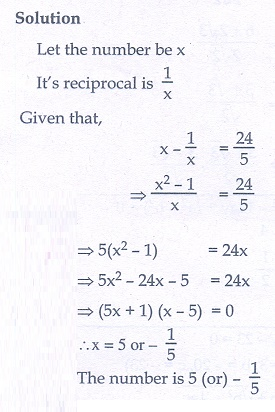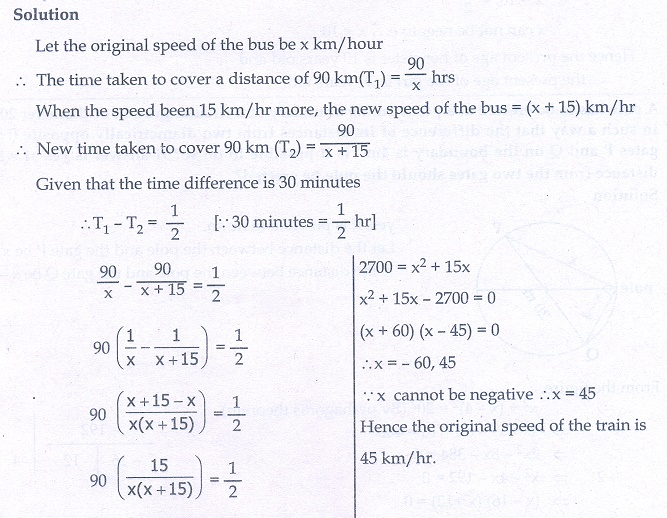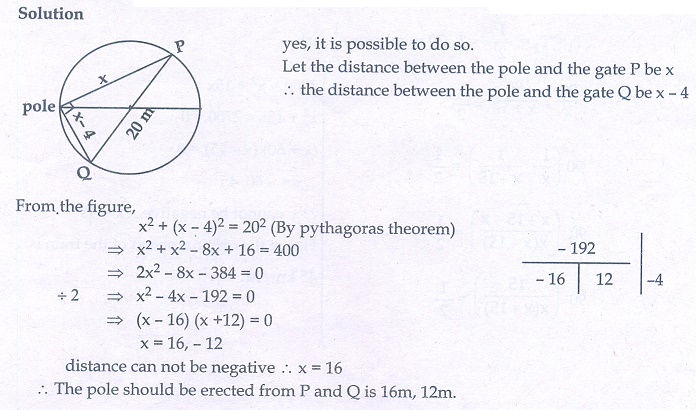Home | | Maths 10th Std | Exercise 3.12: Solving Problems Involving Quadratic Equations

# Exercise 3.12: Solving Problems Involving Quadratic Equations

Maths Book back answers and solution for Exercise questions - Mathematics : Algebra: Solving Problems Involving Quadratic Equations: Exercise Problem Questions with Answer

Exercise 3.12

1. If the difference between a number and its reciprocal is 24/5 , find the number.2. A garden measuring 12m by 16m is to have a pedestrian pathway that is ‘w’ meters wide installed all the way around so that it increases the total area to 285 m2. What is the width of the pathway?3. A bus covers a distance of 90 km at a uniform speed. Had the speed been 15 km/hour more it would have taken 30 minutes less for the journey. Find the original speed of the bus.4. A girl is twice as old as her sister. Five years hence, the product of their ages (in years) will be 375. Find their present ages5. A pole has to be erected at a point on the boundary of a circular ground of diameter 20 m in such a way that the difference of its distances from two diametrically opposite fixed gates P and Q on the boundary is 4 m. Is it possible to do so? If answer is yes at what distance from the two gates should the pole be erected?6. From a group of 2x2 black bees , square root of half of the group went to a tree. Again eight-ninth of the bees went to the same tree. The remaining two got caught up in a fragrant lotus. How many bees were there in total?7. Music is been played in two opposite galleries with certain group of people. In the first gallery a group of 4 singers were singing and in the second gallery 9 singers were singing. The two galleries are separated by the distance of 70 m. Where should a person stand for hearing the same intensity of the singers voice? (Hint: The ratio of the sound intensity is equal to the square of the ratio of their corresponding distances).8. There is a square field whose side is 10 m. A square flower bed is prepared in its centre leaving a gravel path all round the flower bed. The total cost of laying the flower bed and gravelling the path at ₹3 and ₹4 per square metre respectively is ₹364. Find the width of the gravel path.9. Two women together took 100 eggs to a market, one had more than the other. Both sold them for the same sum of money. The first then said to the second: “If I had your eggs, I would have earned ₹15”, to which the second replied: “If I had your eggs, I would have earned ₹ 6 (2/3)”. How many eggs did each had in the beginning?10. The hypotenuse of a right angled triangle is 25 cm and its perimeter 56 cm. Find the length of the smallest side.1. 5, - 1/

2. 1.5 m

3. 45 km/hr

4. 20 years, 10 years

5.Yes, 12 m, 16 m

6. 72

7. 28 m, 42 m

8. 2 m

9. 40, 60

Tags : Problem Questions with Answer, Solution | Mathematics , 10th Mathematics : UNIT 3 : Algebra
Study Material, Lecturing Notes, Assignment, Reference, Wiki description explanation, brief detail
10th Mathematics : UNIT 3 : Algebra : Exercise 3.12: Solving Problems Involving Quadratic Equations | Problem Questions with Answer, Solution | Mathematics